NABARD Manager Quant Aptitude Mock Test 9

# NABARD Manager Quant Aptitude Mock Test 9 - Bank Exams

Test Description

## 20 Questions MCQ Test NABARD Grade B (Manager) Mock Tests Series - NABARD Manager Quant Aptitude Mock Test 9

NABARD Manager Quant Aptitude Mock Test 9 for Bank Exams 2023 is part of NABARD Grade B (Manager) Mock Tests Series preparation. The NABARD Manager Quant Aptitude Mock Test 9 questions and answers have been prepared according to the Bank Exams exam syllabus.The NABARD Manager Quant Aptitude Mock Test 9 MCQs are made for Bank Exams 2023 Exam. Find important definitions, questions, notes, meanings, examples, exercises, MCQs and online tests for NABARD Manager Quant Aptitude Mock Test 9 below.
Solutions of NABARD Manager Quant Aptitude Mock Test 9 questions in English are available as part of our NABARD Grade B (Manager) Mock Tests Series for Bank Exams & NABARD Manager Quant Aptitude Mock Test 9 solutions in Hindi for NABARD Grade B (Manager) Mock Tests Series course. Download more important topics, notes, lectures and mock test series for Bank Exams Exam by signing up for free. Attempt NABARD Manager Quant Aptitude Mock Test 9 | 20 questions in 12 minutes | Mock test for Bank Exams preparation | Free important questions MCQ to study NABARD Grade B (Manager) Mock Tests Series for Bank Exams Exam | Download free PDF with solutions
 1 Crore+ students have signed up on EduRev. Have you?
NABARD Manager Quant Aptitude Mock Test 9 - Question 1

### Size of a tile is 9 inches by 9 inches. The number of tiles needed to cover a floor of 12 feet by 12 feet is

Detailed Solution for NABARD Manager Quant Aptitude Mock Test 9 - Question 1

Length of floor = 12 feet
Breath of floor = 12 feet
So Area of floor = 12 x 12 = 144 feet
Area of tile = 9/12 x 9/12 = 9/16 feet
So the number of tile = 144 9/16 = 256 tiles

NABARD Manager Quant Aptitude Mock Test 9 - Question 2

### The average age of a class of 20 students is 20 years. If the teacher's age is also included the average age increases by one year. The teacher's age is

NABARD Manager Quant Aptitude Mock Test 9 - Question 3

### The average age of A and B is 20 yrs,that of B and C is 19 yrs and that of A and C is 21 yrs. What is the age (in years) of B?

Detailed Solution for NABARD Manager Quant Aptitude Mock Test 9 - Question 3
Total age of A and B = 40
Total age of B and C = 38
Total age of A and C = 42
2A + 2B + 2C = 40 + 38 + 42
2(A + B + C) = 120
A + B + C = 60
B + 42 = 60
∴ Age of B = 60 - 42 = 18
NABARD Manager Quant Aptitude Mock Test 9 - Question 4

Twenty litres of a mixture contains milk and water in the ratio of 5:3. If four litres of this mixture is replaced by 4 litres of milk, then the ratio of the milk to that of the water in the new mixture will be

Detailed Solution for NABARD Manager Quant Aptitude Mock Test 9 - Question 4

Milk in twenty litres of a mixture = 5 /8 x20 = 12.5 lit
Water in four litres of a mixture = 5 /8 x 4 = 2.5 lit
Milk in new mixture = 12.5 - 2.5 + 4 = 14 lit
water in this mixture = 6 lit
∴ Ratio = 14 6 = 7 : 3

NABARD Manager Quant Aptitude Mock Test 9 - Question 5

A sum of money is distributed amongst A,B and C in the proportion 1 2 , 1 3 and 1 4 respectively. A gets Rs 4,000 more than that of B. The sum of money to be distributed was

Detailed Solution for NABARD Manager Quant Aptitude Mock Test 9 - Question 5

LCM (2, 3, 4) = 12
⇒ 1/ 2 : 1 /3 : 1 /4 = 6 : 4 : 3
Also,
A = B + 4000
⇒ 6x - 4x = 4000
⇒ 2x = 4000
⇒ x = 2000
Total money = 6x + 4x + 3x = 13x = 13(2000)
= Rs 26000

NABARD Manager Quant Aptitude Mock Test 9 - Question 6
Choose the correct alternative that will continue the same pattern.
10, 34, 12, 31, 14, 28, 16,....?
Detailed Solution for NABARD Manager Quant Aptitude Mock Test 9 - Question 6
Two patterns alternate here. The first pattern begins with 10 and adds 2 to each number to arrive at the next; the alternating pattern begins with 34 and subtracts 3 each time.
NABARD Manager Quant Aptitude Mock Test 9 - Question 7
Weights of two friends Ram and Shyam are in the ratio of 4:5. Ram's weight increases by 10% and the total weight of Ram and Shyam together becomes 82.8 kg, with an increase of 15%. By what % did the weight of Shyam increase?
NABARD Manager Quant Aptitude Mock Test 9 - Question 8

A sum of money becomes 41/ 40 of itself in 1 4 year at a certain rate of simple interest. The rate of interest per annum is

Detailed Solution for NABARD Manager Quant Aptitude Mock Test 9 - Question 8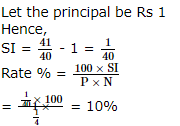NABARD Manager Quant Aptitude Mock Test 9 - Question 9
The probability that a man will live another 10 years is 1/4 and the probability that his wife will live another 10 years is 1/3. Then the probability that neither will be alive in 10 years is
NABARD Manager Quant Aptitude Mock Test 9 - Question 10
A man sells an article at 5% profit. If he had bought it at 5% less and sold it for Rs 1 less, he would have gained 10%. The cost price of the article is
NABARD Manager Quant Aptitude Mock Test 9 - Question 11
20 women can do a work in 16 days. 16 men can complete the same work in fifteen days. What is the ratio between the capacity of a man and a woman?
NABARD Manager Quant Aptitude Mock Test 9 - Question 12
The radii of two right circular cylinder are in ratio 2: 3 and their heights are in ratio 5:4. The ratio of their curved surface areas is
NABARD Manager Quant Aptitude Mock Test 9 - Question 13

In the following questions two equations numbered I and II are given. You have to solve both the equations and give answer.

I. 6p2 + 5p + 1 = 0
II. 20q2 + 9q = - 1

Detailed Solution for NABARD Manager Quant Aptitude Mock Test 9 - Question 13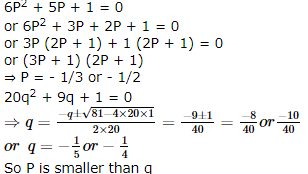NABARD Manager Quant Aptitude Mock Test 9 - Question 14

In the following questions two equations numbered I and II are given. You have to solve both the equations and give answer.

I. 3p2 + 2p - 1 = 0
II. 2q2 + 7q + 6 = 0

Detailed Solution for NABARD Manager Quant Aptitude Mock Test 9 - Question 14

3P2 + 2P - 1 = 0
or 3P2 + 3P - P - 1 = 0
or 3P (P + 1) - 1(P + 1) = 0
or (P + 1) (3P - 1) = 0
or P = - 1, 1/3
2q2 + 7q + 6 = 0
or 2q2 + 4q + 3q + 6 = 0
or 2q (q + 2) + 3 (q + 2) = 0
or (2q + 3) (q + 2) = 0
or q = - 9/2 or q = - 2
∴ P is greater than q

NABARD Manager Quant Aptitude Mock Test 9 - Question 15

The graph shows the number of deaths from road accidents occured during rainy season of 1992.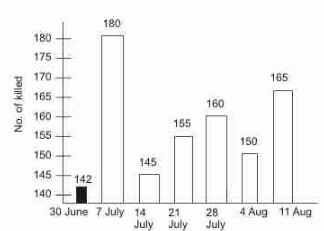Between which two consecutive weeks was the rise in the number of deaths greatest ?

NABARD Manager Quant Aptitude Mock Test 9 - Question 16

The graph shows the number of deaths from road accidents occured during rainy season of 1992.In how many weeks were there more than 150 deaths ?

NABARD Manager Quant Aptitude Mock Test 9 - Question 17

Study the given graphs and table, and answer the following questions given below.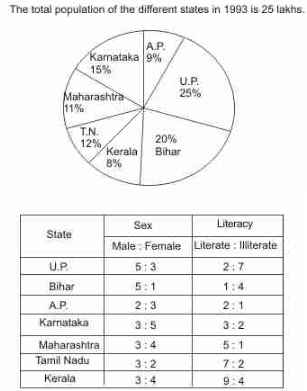Approximately what is the total number of iterate people in Maharastra and Karnataka together ?

NABARD Manager Quant Aptitude Mock Test 9 - Question 18

Study the given graphs and table, and answer the following questions given below.Approximately what will be the percentage of the total male in U.P., Maharastra and Kerala of the total population of the given states ?

NABARD Manager Quant Aptitude Mock Test 9 - Question 19

Marks obtained by six students in different subjects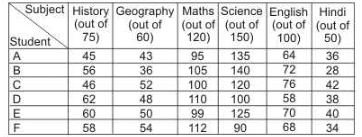What is the average percentage of marks obtained by the students in Science ? (rounded off to be the nearest integer)

NABARD Manager Quant Aptitude Mock Test 9 - Question 20

Marks obtained by six students in different subjectsWhat is the overall percentage of marks obtained by 'B' in all subjects together (rounded off to two digits after decimal) ?

## NABARD Grade B (Manager) Mock Tests Series

40 docs|88 tests
Information about NABARD Manager Quant Aptitude Mock Test 9 Page
In this test you can find the Exam questions for NABARD Manager Quant Aptitude Mock Test 9 solved & explained in the simplest way possible. Besides giving Questions and answers for NABARD Manager Quant Aptitude Mock Test 9, EduRev gives you an ample number of Online tests for practice

40 docs|88 tests